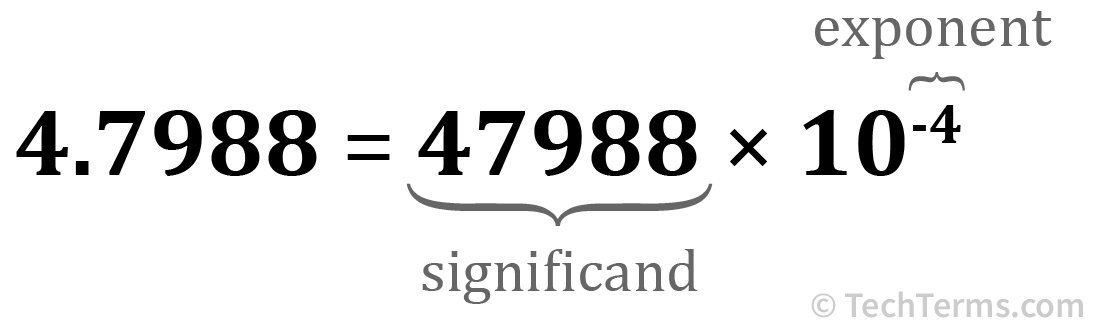# Floating Point

Floating-point numbers are a data type used in computer programming that stores a number with a floating decimal point. A decimal point "floats" when its position is not fixed in place by the number format. For example, 3.145, 12.99, and 234.9876 are all floating-point numbers since the decimal point is not always in the same position.

A computer stores a floating-point number by breaking it into two parts. The first is called the "significand" or "mantissa," which stores the significant digits as a whole number value without a decimal point. The second is the "exponent," which modifies the magnitude of the significand by setting the position of the decimal point. Multiplying the significand by the exponent produces the final value. Some floating-point number formats use a base-10 exponent, while others use a base-2 exponent. For example, storing the number 4.7988 as a floating-point number would use the significand "47988" and the exponent 10-4.The floating-point number "4.7988" represented by its significand and its exponent

Computer programming languages store numbers without decimal points (called integers) as a separate number format. Some languages also support fixed-point numbers, which fix the decimal point in the same place for every number — for example, currency values that always have two digits after a decimal point. Mathematical calculations using integers or fixed-point numbers are less computationally expensive, so using those options when possible is more efficient than using floating-point numbers for everything.

NOTE: Older computer CPUs like the Intel 80386 and the Motorola 68000 series lacked hardware support for floating-point operations, slowly performing them in software instead. These chips used a companion processor, called a floating point unit (FPU) or "math coprocessor," to take over floating-point operations and significantly improve performance. Modern processors include support for floating-point operations without a coprocessor.

##Test Your Knowledge

What contains 1,208,925,819,614,629,174,706,176 bytes?

A
1 pebibyte
0%
B
1 exbibyte
0%
C
1 yobibyte
0%
D
1 zebibyte
0%
Correct! Incorrect!     View the Yobibyte definition.
More Quizzes →

## The Tech Terms Computer Dictionary

The definition of Floating Point on this page is an original definition written by the TechTerms.com team. If you would like to reference this page or cite this definition, please use the green citation links above.

The goal of TechTerms.com is to explain computer terminology in a way that is easy to understand. We strive for simplicity and accuracy with every definition we publish. If you have feedback about this definition or would like to suggest a new technical term, please contact us.